### Altair Silhouette 2Hover over the thumbnail for a full-size version.

Author ]{NO3 author:]{no3 bitesized n-art non-playable rated 2007-11-19 2007-11-20 4 by 22 people. \$Altair Silhouette 2#]{NO3#none#00000000000000000000000000000000000000000000000000000000000000000000000000000000000000000000000000000000000000000000000000000000000000000000000000000000000000000000000000000000000000000000000000000000000000000000000000000000000000000000000000000000000000000000000000000000000000000000000000000000000000000000000000000000000000000000000000000000000000000000000000000000000000000000000000000000000000000000000000000000000000000000000000000000000000000000000000000000000000000000000000000000000000000000000000000000000000000000000000000000000000000000000000000000000000000000000000000000000000000000000000000000000000000000000000000000000000000000000000000000000000000000000000000000000000000000000000000000000000000|12^362,126!12^363,130!12^364,136!12^363,137!12^363,141!12^367,143!12^372,141!12^373,138!12^373,132!12^374,129!12^375,126!12^376,123!12^377,121!12^378,118!12^379,112!12^381,108!12^385,104!12^386,99!12^391,97!12^393,93!12^394,88!12^393,95!12^394,103!12^395,109!12^398,111!12^403,111!12^406,109!12^407,107!12^410,109!12^414,110!12^417,112!12^419,115!12^422,117!12^423,119!12^425,122!12^426,125!12^426,130!12^426,134!12^425,138!12^426,143!12^425,147!12^424,152!12^423,156!12^421,159!12^419,163!12^419,166!12^417,171!12^417,175!12^418,179!12^415,181!12^411,183!12^407,185!12^401,185!12^399,185!12^398,182!12^394,180!12^389,178!12^385,177!12^382,176!12^378,179!12^380,185!12^383,187!12^387,187!12^390,189!12^394,189!12^398,189!12^405,189!12^401,191!12^399,193!12^395,195!12^394,197!12^366,147!12^366,151!12^367,156!12^367,161!12^369,164!12^369,167!12^370,169!12^371,173!12^369,177!12^374,175!12^373,178!12^397,139!12^393,135!12^394,129!12^399,127!12^405,127!12^406,129!12^409,134!12^407,137!12^405,138!12^411,138!12^414,139!12^416,143!12^414,149!12^411,153!12^407,155!12^402,156!12^399,153!12^398,149!12^393,145!12^401,149!12^405,148!12^396,158!12^400,163!12^405,160!12^384,150!12^378,156!12^384,156!12^390,156!12^390,150!12^390,144!12^384,144!12^378,144!12^378,150!12^396,150!12^397,154!12^393,140!12^398,139!12^390,138!12^384,138!12^378,138!12^378,132!12^384,132!12^384,126!12^378,126!12^384,120!12^384,114!12^378,120!12^384,108!12^390,108!12^390,102!12^390,114!12^390,120!12^390,126!12^390,132!12^372,144!12^372,150!12^372,156!12^372,162!12^378,162!12^384,162!12^390,162!12^396,162!12^396,168!12^390,168!12^384,168!12^378,168!12^372,168!12^378,174!12^384,174!12^390,174!12^396,174!12^402,174!12^402,180!12^402,168!12^408,168!12^408,162!12^408,174!12^408,180!12^414,174!12^414,162!12^414,168!12^414,156!12^405,165!12^420,156!12^420,150!12^420,144!12^420,138!12^420,132!12^420,126!12^420,120!12^414,114!12^414,120!12^414,126!12^414,132!12^414,138!12^408,132!12^408,126!12^408,114!12^408,120!12^402,114!12^402,120!12^396,120!12^396,114!12^402,126!12^396,126!12^371,59!12^371,55!12^372,51!12^373,47!12^374,50!12^374,55!12^373,70!12^373,73!12^377,67!12^379,69!12^380,74!12^380,78!12^381,83!12^378,88!12^374,93!12^376,84!12^386,80!12^389,80!12^393,78!12^387,75!12^390,71!12^386,71!12^388,67!12^392,63!12^390,58!12^385,66!12^383,65!12^372,102!12^371,105!12^370,109!12^369,113!12^369,117!12^406,77!12^410,78!12^414,79!12^420,72!12^421,69!12^425,92!12^427,96!12^428,98!12^431,109!12^359,164!12^361,168!12^363,172!12^367,177!12^359,176!12^355,172!12^354,169!12^355,177!12^363,178!12^353,184!12^350,182!12^347,179!12^346,177!12^344,172!12^344,169!12^344,165!12^340,168!12^337,168!12^349,160!12^334,167!12^330,167!12^328,170!12^324,171!12^321,173!12^319,175!12^319,177!12^317,182!12^313,186!12^309,187!12^308,186!12^311,189!12^312,193!12^314,198!12^315,203!12^317,205!12^322,205!12^323,201!12^321,197!12^321,194!12^320,187!12^321,189!12^291,195!12^292,198!12^295,200!12^305,202!12^306,202!12^301,199!12^299,197!12^306,193!12^306,194!12^305,193!12^295,193!12^298,191!12^299,187!12^301,185!12^306,179!12^309,177!12^389,201!12^386,202!12^384,200!12^379,197!12^376,199!12^372,202!12^369,205!12^364,205!12^361,207!12^359,209!12^344,194!12^342,189!12^339,183!12^334,186!12^335,191!12^338,197!12^342,187!12^343,199!12^354,214!12^357,218!12^362,219!12^366,220!12^366,223!12^370,226!12^375,227!12^379,229!12^383,230!12^386,231!12^392,237!12^392,240!12^363,222!12^358,220!12^354,219!12^350,219!12^347,218!12^343,217!12^338,215!12^336,218!12^334,221!12^333,219!12^331,216!12^329,213!12^326,212!12^323,211!12^319,210!12^316,210!12^324,198!12^324,192!12^324,186!12^324,174!12^324,180!12^318,180!12^330,174!12^330,180!12^330,186!12^330,192!12^330,198!12^330,204!12^330,210!12^324,204!12^324,210!12^336,210!12^336,216!12^336,204!12^336,198!12^334,195!12^336,180!12^336,174!12^336,168!12^342,174!12^342,180!12^342,204!12^342,210!12^342,213!12^348,210!12^347,214!12^348,204!12^348,198!12^348,192!12^348,186!12^346,183!12^354,186!12^354,192!12^354,198!12^354,204!12^354,210!12^360,204!12^360,192!12^360,198!12^360,186!12^360,180!12^355,181!12^366,180!12^366,186!12^366,192!12^366,198!12^366,204!12^372,198!12^372,192!12^372,186!12^372,180!12^378,186!12^378,192!12^384,192!12^390,192!12^389,196!12^384,196!12^373,212!12^377,215!12^379,219!12^389,221!12^387,218!12^385,216!12^383,213!12^391,228!12^394,229!12^394,226!12^395,221!12^395,218!12^397,232!12^399,230!12^402,229!12^413,227!12^417,225!12^424,221!12^426,219!12^430,218!12^433,217!12^438,215!12^446,213!12^449,213!12^451,211!12^454,211!12^458,210!12^461,207!12^410,221!12^413,218!12^427,212!12^430,211!12^433,211!12^435,210!12^439,208!12^446,206!12^466,205!12^469,203!12^473,202!12^478,200!12^481,198!12^483,196!12^487,193!12^491,191!12^493,189!12^401,213!12^335,227!12^338,227!12^315,216!12^313,220!12^312,215!12^305,214!12^300,211!12^296,210!12^297,216!12^299,219!12^302,223!12^307,221!12^313,226!12^312,231!12^309,231!12^307,227!12^303,229!12^300,234!12^299,240!12^302,245!12^305,237!12^309,239!12^311,243!12^306,249!12^312,238!12^309,235!12^306,232!12^313,248!12^319,247!12^319,242!12^311,251!12^312,254!12^312,257!12^307,254!12^310,259!12^310,265!12^314,265!12^313,261!12^316,269!12^315,273!12^315,279!12^314,285!12^313,292!12^318,295!12^321,289!12^319,294!12^313,271!12^311,275!12^307,277!12^303,276!12^324,281!12^325,278!12^327,273!12^329,271!12^302,282!12^301,285!12^301,291!12^298,295!12^294,294!12^292,291!12^290,286!12^287,282!12^287,281!12^294,299!12^292,305!12^287,306!12^284,307!12^285,299!12^285,294!12^284,291!12^284,313!12^286,318!12^281,321!12^278,319!12^277,315!12^274,313!12^273,309!12^273,304!12^274,301!12^273,321!12^276,324!12^279,329!12^283,333!12^287,330!12^288,326!12^288,323!12^281,338!12^278,341!12^274,343!12^269,343!12^268,347!12^267,349!12^265,353!12^265,357!12^266,360!12^266,363!12^265,366!12^265,371!12^263,376!12^259,379!12^257,379!12^250,374!12^257,382!12^255,386!12^251,390!12^246,389!12^247,385!12^245,389!12^252,393!12^249,396!12^249,401!12^247,406!12^243,405!12^246,409!12^245,413!12^244,418!12^241,421!12^239,424!12^231,426!12^238,427!12^238,430!12^239,434!12^239,437!12^238,442!12^237,447!12^238,451!12^238,453!12^238,457!12^234,458!12^235,460!12^235,463!12^231,465!12^229,465!12^227,460!12^230,450!12^233,448!12^236,470!12^234,472!12^231,475!12^226,477!12^226,481!12^230,479!12^233,479!12^234,482!12^233,485!12^231,489!12^239,481!12^241,483!12^241,487!12^241,490!12^238,495!12^242,498!12^239,502!12^239,503!12^231,500!12^232,502!12^230,504!12^230,506!12^230,510!12^235,512!12^238,509!12^231,517!12^233,521!12^237,523!12^241,525!12^246,526!12^249,523!12^226,520!12^253,519!12^253,515!12^253,511!12^257,508!12^262,509!12^264,507!12^264,503!12^263,499!12^262,494!12^260,490!12^258,488!12^256,483!12^258,477!12^260,474!12^261,471!12^260,477!12^266,468!12^270,471!12^273,468!12^271,466!12^267,463!12^267,460!12^269,455!12^269,451!12^273,447!12^278,450!12^278,453!12^278,456!12^277,447!12^274,443!12^275,438!12^278,433!12^277,430!12^279,434!12^277,425!12^281,423!12^286,425!12^287,423!12^285,420!12^282,416!12^283,410!12^285,407!12^286,402!12^289,399!12^290,395!12^291,391!12^295,385!12^297,380!12^298,375!12^299,371!12^301,366!12^301,361!12^302,357!12^302,351!12^305,350!12^305,347!12^307,345!12^315,325!12^314,329!12^313,333!12^311,336!12^309,342!12^310,339!12^319,322!12^320,318!12^319,315!12^317,313!12^319,308!12^317,305!12^319,299!12^288,366!12^282,372!12^288,372!12^294,372!12^294,366!12^294,360!12^288,360!12^282,360!12^282,366!12^288,348!12^282,354!12^288,354!12^294,354!12^294,348!12^294,342!12^288,342!12^282,342!12^282,348!12^300,330!12^294,336!12^300,336!12^306,336!12^306,330!12^306,324!12^300,324!12^294,324!12^294,330!12^300,312!12^294,318!12^300,318!12^306,318!12^306,312!12^306,306!12^300,306!12^294,306!12^294,312!12^288,312!12^288,318!12^288,330!12^288,336!12^282,336!12^300,342!12^306,342!12^300,348!12^300,354!12^297,363!12^297,367!12^312,330!12^312,318!12^312,324!12^317,318!12^312,312!12^312,306!12^312,300!12^312,294!12^314,301!12^306,300!12^300,300!12^297,301!12^306,294!12^306,288!12^306,282!12^308,282!12^308,286!12^301,295!12^309,289!12^311,279!12^276,348!12^276,360!12^276,348!12^276,354!12^270,354!12^270,348!12^270,360!12^276,366!12^270,366!12^270,372!12^276,372!12^276,384!12^270,390!12^276,390!12^282,390!12^282,384!12^282,378!12^276,378!12^270,378!12^270,384!12^276,402!12^270,408!12^276,408!12^282,408!12^282,402!12^282,396!12^276,396!12^270,396!12^270,402!12^258,402!12^252,408!12^258,408!12^264,408!12^264,402!12^264,396!12^258,396!12^252,396!12^252,402!12^258,420!12^252,426!12^258,426!12^264,426!12^264,420!12^264,414!12^258,414!12^252,414!12^252,420!12^270,420!12^264,426!12^270,426!12^276,426!12^276,420!12^276,414!12^270,414!12^264,414!12^264,420!12^246,438!12^240,444!12^246,444!12^252,444!12^252,438!12^252,432!12^246,432!12^240,432!12^240,438!12^264,438!12^258,444!12^264,444!12^270,444!12^270,438!12^270,432!12^264,432!12^258,432!12^258,438!12^252,456!12^246,462!12^252,462!12^258,462!12^258,456!12^258,450!12^252,450!12^246,450!12^246,456!12^246,474!12^240,480!12^246,480!12^252,480!12^252,474!12^252,468!12^246,468!12^240,468!12^240,474!12^258,468!12^264,462!12^264,456!12^264,456!12^264,450!12^240,450!12^240,456!12^240,462!12^240,426!12^246,426!12^246,420!12^258,390!12^264,390!12^264,384!12^258,385!12^263,379!12^288,378!12^294,378!12^288,384!12^288,390!12^291,381!12^285,394!12^272,434!12^255,472!12^262,467!12^246,486!12^252,486!12^252,498!12^252,492!12^246,492!12^246,498!12^246,504!12^246,510!12^240,516!12^243,510!12^237,518!12^243,519!12^246,514!12^247,519!12^250,506!12^253,503!12^258,502!12^258,498!12^255,494!12^331,252!12^330,255!12^342,232!12^345,231!12^338,234!12^335,235!12^332,238!12^340,238!12^336,242!12^341,242!12^341,249!12^341,245!12^346,238!12^351,236!12^354,239!12^349,239!12^346,241!12^345,246!12^349,246!12^353,246!12^346,252!12^351,253!12^354,253!12^355,255!12^358,250!12^359,248!12^362,250!12^364,243!12^369,247!12^366,251!12^346,259!12^346,257!12^342,255!12^341,257!12^338,259!12^336,261!12^337,275!12^343,276!12^374,249!12^377,252!12^380,255!12^383,258!12^387,260!12^393,262!12^395,259!12^395,255!12^395,249!12^393,240!12^393,238!12^398,266!12^402,267!12^408,270!12^416,274!12^421,277!12^426,279!12^430,281!12^437,285!12^443,287!12^454,291!12^462,294!12^470,294!12^470,291!12^466,255!12^466,259!12^466,266!12^466,269!12^450,271!12^447,270!12^445,268!12^367,256!12^370,259!12^374,265!12^379,269!12^383,271!12^386,273!12^389,276!12^392,278!12^392,281!12^392,287!12^392,289!12^392,293!12^392,297!12^398,281!12^401,285!12^406,287!12^411,289!12^414,290!12^417,292!12^422,294!12^426,296!12^429,297!12^433,299!12^434,302!12^434,306!12^437,305!12^441,305!12^443,309!12^447,306!12^450,308!12^453,310!12^457,313!12^460,314!12^464,315!12^466,317!12^469,318!12^474,320!12^478,322!12^481,323!12^484,325!12^489,326!12^494,326!12^492,324!12^488,320!12^486,318!12^483,314!12^478,312!12^474,309!12^469,310!12^466,309!12^463,306!12^460,304!12^456,301!12^452,299!12^447,296!12^443,294!12^437,294!12^435,291!12^431,290!12^426,289!12^423,286!12^418,283!12^414,282!12^410,279!12^405,277!12^402,275!12^399,275!12^398,273!12^395,271!12^392,270!12^390,266!12^370,252!12^371,257!12^374,261!12^377,266!12^383,268!12^386,266!12^382,263!12^379,260!12^376,257!12^374,255!12^391,273!12^398,275!12^385,270!12^403,281!12^408,282!12^411,285!12^414,287!12^420,290!12^425,292!12^429,294!12^433,295!12^436,297!12^439,300!12^442,299!12^446,301!12^451,302!12^454,305!12^458,307!12^462,310!12^466,313!12^472,315!12^475,315!12^479,318!12^482,319!12^481,302!12^483,303!12^486,305!12^489,306!12^498,304!12^499,307!12^501,313!12^501,317!12^499,321!12^498,295!12^498,288!12^498,285!12^498,281!12^498,277!12^498,274!12^501,270!12^501,266!12^501,261!12^503,258!12^505,245!12^504,253!12^508,252!12^511,249!12^511,244!12^513,243!12^513,239!12^513,237!12^514,234!12^511,255!12^510,259!12^511,265!12^517,266!12^519,262!12^521,260!12^522,262!12^524,266!12^522,267!12^522,271!12^523,277!12^525,280!12^526,283!12^527,288!12^529,290!12^532,292!12^531,294!12^531,299!12^531,303!12^534,309!12^536,311!12^538,313!12^542,313!12^545,313!12^543,317!12^548,320!12^551,318!12^545,324!12^541,327!12^537,329!12^533,331!12^531,334!12^535,325!12^536,321!12^539,318!12^526,337!12^525,335!12^523,334!12^521,331!12^519,327!12^517,323!12^516,317!12^514,313!12^513,311!12^511,307!12^510,303!12^509,300!12^507,298!12^506,295!12^505,291!12^503,287!12^510,276!12^504,282!12^510,282!12^516,282!12^516,276!12^516,270!12^510,270!12^504,270!12^504,276!12^504,264!12^508,260!12^507,265!12^522,276!12^522,282!12^516,294!12^510,300!12^516,300!12^522,300!12^522,294!12^522,288!12^516,288!12^510,288!12^510,294!12^528,294!12^528,300!12^522,312!12^516,318!12^522,318!12^528,318!12^528,312!12^528,306!12^522,306!12^516,306!12^516,312!12^522,324!12^528,324!12^534,318!12^534,312!12^528,330!12^522,330!12^534,324!12^489,206!12^486,209!12^483,202!12^487,217!12^487,221!12^495,225!12^488,229!12^487,231!12^490,238!12^487,239!12^494,244!12^489,250!12^487,254!12^490,256!12^491,265!12^496,259!12^496,254!12^381,286!12^378,282!12^374,279!12^531,339!12^533,338!12^537,338!12^539,338!12^542,337!12^545,335!12^547,334!12^549,338!12^554,338!12^558,337!12^561,335!12^569,335!12^565,337!12^559,329!12^562,327!12^583,327!12^581,327!12^575,326!12^585,334!12^587,337!12^588,339!12^582,334!12^579,334!12^549,343!12^551,345!12^553,350!12^555,355!12^559,357!12^561,358!12^565,361!12^560,349!12^561,345!12^565,343!12^566,353!12^567,350!12^572,349!12^570,356!12^574,357!12^575,355!12^587,347!12^589,347!12^592,348!12^591,352!12^589,357!12^591,362!12^590,363!12^561,366!12^558,366!12^554,364!12^554,361!12^550,363!12^547,367!12^551,372!12^547,371!12^555,376!12^559,377!12^563,375!12^569,373!12^572,374!12^574,375!12^577,376!12^584,376!12^589,377!12^590,374!12^590,378!12^590,385!12^590,390!12^568,382!12^566,382!12^570,388!12^575,387!12^574,391!12^572,394!12^571,395!12^565,390!12^563,388!12^560,388!12^557,387!12^553,384!12^552,381!12^562,395!12^565,392!12^558,391!12^557,394!12^557,397!12^552,387!12^548,388!12^549,393!12^549,399!12^551,405!12^554,407!12^557,409!12^560,409!12^561,406!12^567,403!12^571,403!12^574,403!12^580,404!12^586,405!12^589,406!12^589,407!12^570,409!12^568,409!12^573,418!12^571,417!12^563,419!12^563,422!12^568,424!12^565,425!12^560,424!12^556,424!12^553,421!12^556,417!12^558,416!12^554,413!12^555,427!12^557,429!12^561,431!12^563,430!12^549,422!12^547,425!12^547,429!12^550,433!12^553,435!12^556,437!12^558,439!12^555,441!12^549,440!12^565,437!12^568,438!12^570,440!12^573,435!12^575,435!12^579,434!12^583,435!12^586,436!12^589,437!12^584,439!12^591,428!12^591,425!12^590,418!12^585,414!12^591,444!12^589,447!12^590,461!12^590,463!12^591,477!12^591,482!12^591,485!12^588,485!12^584,465!12^582,466!12^579,466!12^575,463!12^572,461!12^567,459!12^565,457!12^559,449!12^550,446!12^550,451!12^545,453!12^545,457!12^543,462!12^547,465!12^551,464!12^550,461!12^547,470!12^551,470!12^555,471!12^555,475!12^559,476!12^550,474!12^550,478!12^553,481!12^555,482!12^559,483!12^562,485!12^565,486!12^569,489!12^573,490!12^576,490!12^578,491!12^570,474!12^566,471!12^561,466!12^560,465!12^557,457!12^579,495!12^578,499!12^575,498!12^572,501!12^571,498!12^569,497!12^567,495!12^574,494!12^571,493!12^563,491!12^559,491!12^555,490!12^552,493!12^552,497!12^553,499!12^551,503!12^551,506!12^551,509!12^550,514!12^553,517!12^558,517!12^558,513!12^564,514!12^565,509!12^566,508!12^567,517!12^571,517!12^573,513!12^574,518!12^577,517!12^579,513!12^572,520!12^570,522!12^566,522!12^563,523!12^559,523!12^556,524!12^552,523!12^561,519!12^555,520!12^549,518!12^543,520!12^545,521!12^543,517!12^541,513!12^539,510!12^537,507!12^535,503!12^535,499!12^534,497!12^534,493!12^533,489!12^533,485!12^534,482!12^537,477!12^539,474!12^541,471!12^542,467!12^540,474!12^540,480!12^540,486!12^540,492!12^540,498!12^540,504!12^552,504!12^546,504!12^546,510!12^543,510!12^547,514!12^546,498!12^546,492!12^546,486!12^546,480!12^546,474!12^537,484!12^535,489!12^552,486!12^550,490!12^558,486!12^541,461!12^538,457!12^541,456!12^544,453!12^542,447!12^541,441!12^542,436!12^539,435!12^539,430!12^540,425!12^539,422!12^539,419!12^539,416!12^538,413!12^538,408!12^537,407!12^535,406!12^535,402!12^535,398!12^535,395!12^535,391!12^546,450!12^546,444!12^546,438!12^546,432!12^541,433!12^540,426!12^546,420!12^546,414!12^540,420!12^540,414!12^540,408!12^546,408!12^552,408!12^552,414!12^549,417!12^540,402!12^540,396!12^540,390!12^546,402!12^546,390!12^546,396!12^546,378!12^552,378!12^546,384!12^540,384!12^540,378!12^540,372!12^540,366!12^540,360!12^540,354!12^540,348!12^540,342!12^546,342!12^546,348!12^546,354!12^546,360!12^545,364!12^544,371!12^550,352!12^550,357!12^531,342!12^533,345!12^531,349!12^529,354!12^529,357!12^529,362!12^529,365!12^529,369!12^530,373!12^531,375!12^534,380!12^534,384!12^534,378!12^534,372!12^534,366!12^534,360!12^534,354!12^534,348!12^398,296!12^402,299!12^405,303!12^409,304!12^411,306!12^415,309!12^419,313!12^411,299!12^409,296!12^384,287!12^380,283!12^376,279!12^373,276!12^423,305!12^426,311!12^430,314!12^434,313!12^431,315!12^439,318!12^441,319!12^444,323!12^446,326!12^449,330!12^452,331!12^456,326!12^460,328!12^463,329!12^463,333!12^468,335!12^469,337!12^473,338!12^477,339!12^479,341!12^481,342!12^484,343!12^400,310!12^397,309!12^392,309!12^389,306!12^384,303!12^380,303!12^377,303!12^373,303!12^368,301!12^364,300!12^359,299!12^355,299!12^353,301!12^349,303!12^346,300!12^345,298!12^342,294!12^341,291!12^342,303!12^339,306!12^335,306!12^333,303!12^333,299!12^331,292!12^332,289!12^334,310!12^333,313!12^329,315!12^328,311!12^327,318!12^327,323!12^326,326!12^328,322!12^332,320!12^336,319!12^339,316!12^344,316!12^347,318!12^347,319!12^351,320!12^351,321!12^357,320!12^359,322!12^361,324!12^364,327!12^367,328!12^368,331!12^368,335!12^366,339!12^364,342!12^365,346!12^361,351!12^358,349!12^358,345!12^354,348!12^353,349!12^351,351!12^347,354!12^343,354!12^341,351!12^341,348!12^342,351!12^340,356!12^338,359!12^334,359!12^331,359!12^333,355!12^334,351!12^404,311!12^406,312!12^408,316!12^410,318!12^413,318!12^417,319!12^419,319!12^421,320!12^424,320!12^428,322!12^431,322!12^433,324!12^436,326!12^438,328!12^440,330!12^442,332!12^445,336!12^448,340!12^450,343!12^450,346!12^450,351!12^454,352!12^458,351!12^462,350!12^464,346!12^469,348!12^470,351!12^471,354!12^465,352!12^465,357!12^468,361!12^470,363!12^473,360!12^478,363!12^482,368!12^480,366!12^472,366!12^474,368!12^475,372!12^479,374!12^479,378!12^480,382!12^482,383!12^482,385!12^482,389!12^482,393!12^484,398!12^484,379!12^486,378!12^485,402!12^486,404!12^491,405!12^495,404!12^495,398!12^498,398!12^501,401!12^504,401!12^501,404!12^500,408!12^504,393!12^502,392!12^497,386!12^496,381!12^495,376!12^506,385!12^508,382!12^507,379!12^506,374!12^507,369!12^503,364!12^502,359!12^500,356!12^499,352!12^496,350!12^494,350!12^491,351!12^487,353!12^484,353!12^481,350!12^478,348!12^476,346!12^488,355!12^489,359!12^493,363!12^496,367!12^498,368!12^501,370!12^503,374!12^503,378!12^504,369!12^499,364!12^498,360!12^495,355!12^492,356!12^495,359!12^504,363!12^385,347!12^380,346!12^382,341!12^383,337!12^386,334!12^390,332!12^394,329!12^399,328!12^401,331!12^396,336!12^392,339!12^391,342!12^385,351!12^388,354!12^393,354!12^394,349!12^396,346!12^397,346!12^396,355!12^401,354!12^401,353!12^402,351!12^403,359!12^406,361!12^410,361!12^409,359!12^408,351!12^411,353!12^413,354!12^415,362!12^418,361!12^401,364!12^402,368!12^407,368!12^413,369!12^418,367!12^411,369!12^406,333!12^408,336!12^407,339!12^412,334!12^416,337!12^415,342!12^418,345!12^419,346!12^420,348!12^423,350!12^426,350!12^430,350!12^434,350!12^439,350!12^443,351!12^441,355!12^438,357!12^434,358!12^432,358!12^444,357!12^449,357!12^452,361!12^450,364!12^453,366!12^455,368!12^461,369!12^461,374!12^459,375!12^461,376!12^464,378!12^467,379!12^468,382!12^471,383!12^471,388!12^469,391!12^468,396!12^466,395!12^463,396!12^460,393!12^458,392!12^455,389!12^451,385!12^446,383!12^444,380!12^441,377!12^439,376!12^439,374!12^438,374!12^438,370!12^439,366!12^442,363!12^443,361!12^436,376!12^434,381!12^431,381!12^427,381!12^424,379!12^423,376!12^426,373!12^423,372!12^420,371!12^426,365!12^377,343!12^377,340!12^377,337!12^377,333!12^372,333!12^369,339!12^369,344!12^374,347!12^378,324!12^372,330!12^378,330!12^384,330!12^384,324!12^384,318!12^378,318!12^372,318!12^372,324!12^396,318!12^390,324!12^396,324!12^402,324!12^402,318!12^402,312!12^396,312!12^390,312!12^390,318!12^390,330!12^384,312!12^378,312!12^372,312!12^372,306!12^378,306!12^384,306!12^360,312!12^354,318!12^360,318!12^366,318!12^366,312!12^366,306!12^360,306!12^354,306!12^354,312!12^366,324!12^348,306!12^342,306!12^346,304!12^336,312!12^342,312!12^348,312!12^408,318!12^408,324!12^408,330!12^414,330!12^414,324!12^420,324!12^420,330!12^420,336!12^420,342!12^432,336!12^426,342!12^432,342!12^438,342!12^438,336!12^438,330!12^432,330!12^426,330!12^426,336!12^426,324!12^432,324!12^426,348!12^432,348!12^438,348!12^444,348!12^444,342!12^444,336!12^446,351!12^456,354!12^450,354!12^456,360!12^462,360!12^462,354!12^462,366!12^456,366!12^468,366!12^468,372!12^474,372!12^474,378!12^474,384!12^474,390!12^474,396!12^480,396!12^480,390!12^480,384!12^372,360!12^366,366!12^372,366!12^378,366!12^378,360!12^378,354!12^372,354!12^366,354!12^366,360!12^372,348!12^366,348!12^378,348!12^384,348!12^384,354!12^384,360!12^384,366!12^390,366!12^390,360!12^396,360!12^402,360!12^396,366!12^360,366!12^360,360!12^360,354!12^354,354!12^354,360!12^348,360!12^354,364!12^348,367!12^350,373!12^358,372!12^355,374!12^350,356!12^344,360!12^347,364!12^345,372!12^341,373!12^336,372!12^331,370!12^333,366!12^336,366!12^330,366!12^328,373!12^324,373!12^323,371!12^323,368!12^323,366!12^316,366!12^316,361!12^317,357!12^317,354!12^318,349!12^319,345!12^319,341!12^319,338!12^319,352!12^360,372!12^366,372!12^372,372!12^378,372!12^384,372!12^390,372!12^396,372!12^402,372!12^408,372!12^414,372!12^420,372!12^414,384!12^408,390!12^414,390!12^420,390!12^420,384!12^420,378!12^414,378!12^408,378!12^408,384!12^396,384!12^390,390!12^396,390!12^402,390!12^402,384!12^402,378!12^396,378!12^390,378!12^390,384!12^378,384!12^372,390!12^378,390!12^384,390!12^384,384!12^384,378!12^378,378!12^372,378!12^372,384!12^378,402!12^372,408!12^378,408!12^384,408!12^384,402!12^384,396!12^378,396!12^372,396!12^372,402!12^396,402!12^390,408!12^396,408!12^402,408!12^402,402!12^402,396!12^396,396!12^390,396!12^390,402!12^414,402!12^408,408!undefined^undefined,undefined!undefined^undefined,undefined!12^420,402!12^420,396!12^414,396!12^408,396!12^408,402!12^426,384!12^432,384!12^438,384!12^438,378!12^432,396!12^426,402!12^432,402!12^438,402!12^438,396!12^438,390!12^432,390!12^426,390!12^426,396!12^444,402!12^444,396!12^444,390!12^444,384!12^446,391!12^449,393!12^453,397!12^456,401!12^459,403!12^464,401!12^450,396!12^450,402!12^456,402!12^419,405!12^424,405!12^458,406!12^459,409!12^462,411!12^466,411!12^454,404!12^471,412!12^473,414!12^475,416!12^478,419!12^493,411!12^496,414!12^496,418!12^494,422!12^498,424!12^495,427!12^499,430!12^501,433!12^502,438!12^502,443!12^500,447!12^498,450!12^492,449!12^490,452!12^490,456!12^490,459!12^489,454!12^488,449!12^486,446!12^483,441!12^481,438!12^481,433!12^480,429!12^479,429!12^475,429!12^473,428!12^476,423!12^473,424!12^471,424!12^468,424!12^468,426!12^465,426!12^462,426!12^461,424!12^462,408!12^468,408!12^464,404!12^468,402!12^474,402!12^480,402!12^480,408!12^474,408!12^486,414!12^480,414!12^486,408!12^492,408!12^492,414!12^486,420!12^492,420!12^480,420!12^480,426!12^486,426!12^492,426!12^492,432!12^486,432!12^486,438!12^492,438!12^498,438!12^498,432!12^486,444!12^492,444!12^498,444!12^508,448!12^508,446!12^510,443!12^510,447!12^508,451!12^511,449!12^453,414!12^448,416!12^443,418!12^439,420!12^435,421!12^430,421!12^425,422!12^449,421!12^453,421!12^428,431!12^429,431!12^433,432!12^436,431!12^441,431!12^445,431!12^448,430!12^453,429!12^432,433!12^428,433!12^415,420!12^411,419!12^409,418!12^412,413!12^414,411!12^415,408!12^413,407!12^408,414!12^402,414!12^396,414!12^390,414!12^400,418!12^400,422!12^396,422!12^393,422!12^389,423!12^386,426!12^396,420!12^390,420!12^384,420!12^384,414!12^385,432!12^391,431!12^395,431!12^400,431!12^403,429!12^406,429!12^411,427!12^416,427!12^418,426!12^408,433!12^409,437!12^405,437!12^404,434!12^401,436!12^398,438!12^390,438!12^396,438!12^390,433!12^396,433!12^381,431!12^377,431!12^371,433!12^373,439!12^379,439!12^386,439!12^378,426!12^383,425!12^378,420!12^378,414!12^372,414!12^372,420!12^372,426!12^366,378!12^360,378!12^366,384!12^360,384!12^354,378!12^348,378!12^342,378!12^336,378!12^330,378!12^330,372!12^324,378!12^321,378!12^319,383!12^316,386!12^314,390!12^312,394!12^305,395!12^306,391!12^307,386!12^308,396!12^305,401!12^305,405!12^308,408!12^309,412!12^311,415!12^314,418!12^318,420!12^322,423!12^326,426!12^329,426!12^333,428!12^337,431!12^349,428!12^344,428!12^343,426!12^339,426!12^336,424!12^333,422!12^329,422!12^326,421!12^323,417!12^326,419!12^321,414!12^319,412!12^317,409!12^318,404!12^319,399!12^321,396!12^325,393!12^329,390!12^331,394!12^333,390!12^337,390!12^343,388!12^345,383!12^341,381!12^336,381!12^333,382!12^330,387!12^326,386!12^325,381!12^324,390!12^318,390!12^322,385!12^316,395!12^312,402!12^314,398!12^309,402!12^313,407!12^313,411!12^316,415!12^348,389!12^353,387!12^355,383!12^349,383!12^359,389!12^363,388!12^368,387!12^368,391!12^366,397!12^361,398!12^359,394!12^356,399!12^354,400!12^350,399!12^343,393!12^343,398!12^343,401!12^341,404!12^338,407!12^336,409!12^346,407!12^349,403!12^354,403!12^354,408!12^351,412!12^348,415!12^346,418!12^350,423!12^355,422!12^354,418!12^354,414!12^360,402!12^366,402!12^366,408!12^360,408!12^360,414!12^366,414!12^366,420!12^360,420!12^360,426!12^366,426!12^366,432!12^360,432!12^354,432!12^354,426!12^342,432!12^348,432!12^354,444!12^348,450!12^354,450!12^360,450!12^360,444!12^360,438!12^354,438!12^348,438!12^348,444!12^354,462!12^348,468!12^354,468!12^360,468!12^360,462!12^360,456!12^354,456!12^348,456!12^348,462!12^354,480!12^348,486!12^354,486!12^360,486!12^360,480!12^360,474!12^354,474!12^348,474!12^348,480!12^354,498!12^348,504!12^354,504!12^360,504!12^360,498!12^360,492!12^354,492!12^348,492!12^348,498!12^372,498!12^366,504!12^372,504!12^378,504!12^378,498!12^378,492!12^372,492!12^366,492!12^366,498!12^372,480!12^366,486!12^372,486!12^378,486!12^378,480!12^378,474!12^372,474!12^366,474!12^366,480!12^372,462!12^366,468!12^372,468!12^378,468!12^378,462!12^378,456!12^372,456!12^366,456!12^366,462!12^390,462!12^384,468!12^390,468!12^396,468!12^396,462!12^396,456!12^390,456!12^384,456!12^384,462!12^390,480!12^384,486!12^390,486!undefined^undefined,undefined!12^396,480!12^396,474!12^390,474!12^384,474!12^384,480!undefined^undefined,undefined!12^390,498!12^384,504!12^390,504!12^396,504!12^396,498!12^396,492!12^390,492!12^384,492!12^384,498!12^390,516!12^384,522!12^390,522!12^396,522!12^396,516!12^396,510!12^390,510!12^384,510!12^384,516!12^372,516!12^366,522!12^372,522!12^378,522!12^378,516!12^378,510!12^372,510!12^366,510!12^366,516!12^336,498!12^330,504!12^336,504!12^342,504!12^342,498!12^342,492!12^336,492!12^330,492!12^330,498!12^336,516!12^330,522!12^336,522!12^342,522!12^342,516!12^342,510!12^336,510!12^330,510!12^330,516!12^348,522!12^348,516!12^348,510!12^354,510!12^360,510!12^360,516!12^360,522!12^353,516!12^355,518!12^356,521!12^358,523!12^358,528!12^356,533!12^354,536!12^349,538!12^346,536!12^346,532!12^346,526!12^366,438!12^366,444!12^366,450!12^372,450!12^384,450!12^378,450!12^372,444!12^378,444!12^384,444!12^390,444!12^390,450!12^396,450!12^396,444!12^398,451!12^399,455!12^399,461!12^400,466!12^400,471!12^401,476!12^403,482!12^404,487!12^406,492!12^410,523!12^410,516!12^409,511!12^408,503!12^407,496!12^408,506!12^411,528!12^411,534!12^411,539!12^411,546!12^410,553!12^409,559!12^408,564!12^408,570!12^406,574!12^407,569!12^402,522!12^402,516!12^402,510!12^402,504!12^402,498!12^402,492!12^399,486!12^395,486!12^408,516!12^408,522!12^360,528!12^366,528!12^372,528!12^378,528!12^384,528!12^390,528!12^396,528!12^408,528!12^402,528!12^366,540!12^360,546!12^366,546!12^372,546!12^372,540!12^372,534!12^366,534!12^360,534!12^360,540!12^384,540!12^378,546!12^384,546!12^390,546!12^390,540!12^390,534!12^384,534!12^378,534!12^378,540!12^402,540!12^396,546!12^402,546!12^408,546!12^408,540!12^408,534!12^402,534!12^396,534!12^396,540!12^366,558!12^360,564!12^366,564!12^372,564!12^372,558!12^372,552!12^366,552!12^360,552!12^360,558!12^384,558!12^378,564!12^384,564!12^390,564!12^390,558!12^390,552!12^384,552!12^378,552!12^378,558!12^402,558!12^396,564!12^402,564!12^408,564!12^408,558!12^408,552!12^402,552!12^396,552!12^396,558!12^348,558!12^342,564!12^348,564!12^354,564!12^354,558!12^354,552!12^348,552!12^342,552!12^342,558!12^336,480!12^330,486!12^336,486!12^342,486!12^342,480!12^342,474!12^336,474!undefined^undefined,undefined!12^330,480!12^331,475!12^333,471!12^334,466!12^336,461!12^337,454!12^337,448!12^339,443!12^340,437!12^342,438!12^342,444!12^342,450!12^342,456!12^342,462!12^342,468!12^336,468!12^338,462!12^339,458!12^354,546!12^354,540!12^348,540!12^348,546!12^342,546!12^342,540!12^342,534!12^342,528!12^336,528!12^330,528!12^330,534!12^336,534!12^336,540!12^330,540!12^330,546!12^330,552!12^332,557!12^332,553!12^331,548!12^332,544!12^331,563!12^331,567!12^336,567!12^338,564!12^339,560!12^402,570!12^396,570!12^384,570!12^390,570!12^378,570!12^372,570!12^360,570!12^366,570!12^354,570!12^342,570!12^348,570!12^336,570!12^336,576!12^342,576!12^354,576!12^348,576!12^360,576!12^366,576!12^372,576!12^378,576!12^384,576!12^390,576!12^396,576!12^402,576!12^330,576!12^330,570!12^324,570!12^324,576!12^318,576!12^318,570!12^312,570!12^312,576!12^318,558!12^312,564!12^318,564!12^324,564!12^324,558!12^324,552!12^318,552!12^312,552!12^312,558!12^318,546!12^324,546!12^324,540!12^324,534!12^324,528!12^324,522!12^324,516!12^324,510!12^324,504!12^324,498!12^327,489!12^323,493!12^323,500!12^320,506!12^315,508!12^313,514!12^311,517!12^310,520!12^309,524!12^306,526!12^310,533!12^316,533!12^316,538!12^316,544!12^313,547!12^306,549!12^303,545!12^304,537!12^306,534!12^319,529!12^321,524!12^318,521!12^314,526!12^309,529!12^314,520!12^318,516!12^318,510!12^303,532!12^299,534!12^308,554!12^308,558!12^309,562!12^322,541!12^321,535!12^328,554!12^328,560!12^308,573!12^309,568!12^309,565!12^300,549!12^298,554!12^297,558!12^300,559!12^292,559!12^292,564!12^292,568!12^289,562!12^284,563!12^286,569!12^283,568!12^278,571!12^275,574!12^276,568!12^279,564!12^283,561!12^284,556!12^287,552!12^290,548!12^294,544!12^298,539!12^301,541!12^295,546!12^298,545!12^292,552!12^295,551!12^290,558!12^288,558!12^294,555!12^295,539!12^291,538!12^288,541!12^286,541!12^283,540!12^283,534!12^284,528!12^286,523!12^287,516!12^288,520!12^283,545!12^286,533!12^288,526!12^292,521!12^294,516!12^298,511!12^298,507!12^293,513!12^293,506!12^293,500!12^294,493!12^317,424!12^315,426!12^313,429!12^312,434!12^311,436!12^310,439!12^310,444!12^309,448!12^308,453!12^307,459!12^304,462!12^303,465!12^301,471!12^299,476!12^298,480!12^296,483!12^296,488!12^306,463!12^306,468!12^306,471!12^305,476!12^304,480!12^302,484!12^302,488!12^299,493!12^299,496!12^299,503!12^298,499!12^296,498!12^294,511!12^298,483!12^301,476!12^304,468!12^321,428!12^321,433!12^319,436!12^318,441!12^317,444!12^316,450!12^315,455!12^315,458!12^314,463!12^314,466!12^312,474!12^311,479!12^309,484!12^293,533!12^294,529!12^298,524!12^301,520!12^303,514!12^305,508!12^308,504!12^312,499!12^313,491!12^315,485!12^319,472!12^319,469!12^323,463!12^323,458!12^348,327!12^346,325!12^343,324!12^338,324!12^334,328!12^327,334!12^328,333!12^341,331!12^341,334!12^340,338!12^346,336!12^346,340!12^346,343!12^352,332!12^352,337!12^358,331!12^359,335!12^418,437!12^421,438!12^423,434!12^414,443!12^416,445!12^418,448!12^424,446!12^424,450!12^426,453!12^427,455!12^432,461!12^432,466!12^429,464!12^434,459!12^434,455!12^436,450!12^434,448!12^431,446!12^432,439!12^435,441!12^440,447!12^444,448!12^439,450!12^439,453!12^449,452!12^454,459!12^454,464!12^450,464!12^443,461!12^442,464!12^443,466!12^438,469!12^436,469!12^439,474!12^444,471!12^449,469!12^455,466!12^459,463!12^461,467!12^463,469!12^466,471!12^459,468!12^454,469!12^456,471!12^448,471!12^444,475!12^448,478!12^452,480!12^453,478!12^454,482!12^456,484!12^461,484!12^463,481!12^461,478!12^457,490!12^457,494!12^459,496!12^464,496!12^466,493!12^463,487!12^466,486!12^471,487!12^478,486!12^474,480!12^474,476!12^469,476!12^466,479!12^476,478!12^482,476!12^480,473!12^480,469!12^480,464!12^479,460!12^476,456!12^476,452!12^476,448!12^475,443!12^475,439!12^482,468!12^482,474!12^487,475!12^491,476!12^494,477!12^496,478!12^496,473!12^496,470!12^499,464!12^500,465!12^503,470!12^504,479!12^506,476!12^508,478!12^507,480!12^509,483!12^510,488!12^511,492!12^478,481!12^483,482!12^476,487!12^469,490!12^465,500!12^468,496!12^471,493!12^476,491!12^479,491!12^481,493!12^486,496!12^484,488!12^488,491!12^492,492!12^496,493!12^499,496!12^501,494!12^503,489!12^501,489!12^498,491!12^496,487!12^493,489!12^490,487!12^490,484!12^497,496!12^469,503!12^471,503!12^474,503!12^479,503!12^482,503!12^467,504!12^470,507!12^472,510!12^476,511!12^478,509!12^485,509!12^490,513!12^493,510!12^493,505!12^490,504!12^488,501!12^491,502!12^495,504!12^499,505!12^500,505!12^496,514!12^501,514!12^503,514!12^499,513!12^496,511!12^479,513!12^483,514!12^484,517!12^486,519!12^489,519!12^493,520!12^494,521!12^496,523!12^487,523!12^484,525!12^481,528!12^479,531!12^474,529!12^474,525!12^472,523!12^479,523!12^481,518!12^483,520!12^477,528!12^478,522!12^471,521!12^472,516!12^471,514!12^464,512!12^467,518!12^467,522!12^461,521!12^459,519!12^474,537!12^478,539!12^483,539!12^487,536!12^491,535!12^491,529!12^494,530!12^498,531!12^501,533!12^494,537!12^496,539!12^497,543!12^497,548!12^498,553!12^498,559!12^498,565!12^503,573!12^502,569!12^501,566!12^493,573!12^490,568!12^487,569!12^484,569!12^480,566!12^487,566!12^491,564!12^490,559!12^487,556!12^483,556!12^482,559!12^478,556!12^474,553!12^471,551!12^472,543!12^476,541!12^479,543!12^479,548!12^476,548!12^483,541!12^482,549!12^486,549!12^492,546!12^492,540!12^486,540!12^486,546!12^499,574!12^498,570!12^492,570!12^492,564!12^492,558!12^492,552!12^503,499!12^507,499!12^509,499!12^512,502!12^513,508!12^514,509!12^514,514!12^514,520!12^514,521!12^510,521!12^506,520!12^509,514!12^507,512!12^512,527!12^509,529!12^506,528!12^515,530!12^517,533!12^517,538!12^519,542!12^519,548!12^516,549!12^515,554!12^517,559!12^517,564!12^518,569!12^521,574!12^514,573!12^513,568!12^513,564!12^512,559!12^512,553!12^511,550!12^509,544!12^506,542!12^505,540!12^511,534!12^511,537!12^514,539!12^514,544!12^517,547!12^452,518!12^448,520!12^449,517!12^448,514!12^444,511!12^448,509!12^446,506!12^448,499!12^443,496!12^440,492!12^435,486!12^431,482!12^427,482!12^424,477!12^424,473!12^420,469!12^413,463!12^416,465!12^449,488!12^444,484! 12^74,520!12^71,515!12^59,514!12^58,518!12^49,516!12^52,525!12^45,522!12^41,519!12^37,526!12^39,535!12^34,538!12^81,517!12^84,524!12^82,531!12^89,537!12^88,551!12^81,556!12^84,562!12^73,567!12^58,568!12^53,562!12^44,564!12^37,560!12^40,553!12^34,550# another silhouette of altair from assassin's creed. http://numa.notdot.net/map/107376 I was dissapointed when it was delayed from release in Australia and New Zealand on the 15th, till the 21st.

## Other maps by this author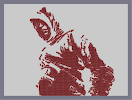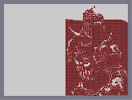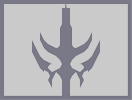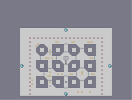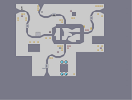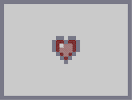Altair Silhouette Shivering Isles Silhouette Daedric Claymore TS channels Network Pixel Heart

Pages: (0)

yup

great

### Nice!

Looks very cool, although the face and the top of the hood look funny. What's that circly thingy at the bottom left corner?

### Brilliant Details!

*agrees with atob tho*

### as atob said

i think the idea isnt the best for this pic - coz the hood instead of looking cool - just makes his hear seem too small and it looks rediculous
and whats that on the bottom left?
3.5/5

### the face

and right arm are very effective. the rest is a bit messy. body seems a little too chubby.

3/5

### well

apparently im the only one who doesnt like it.
okay then. :)

### nice

u should give him some genetic memory symbols
4|5

### meh

2/5
looks sorta blurry